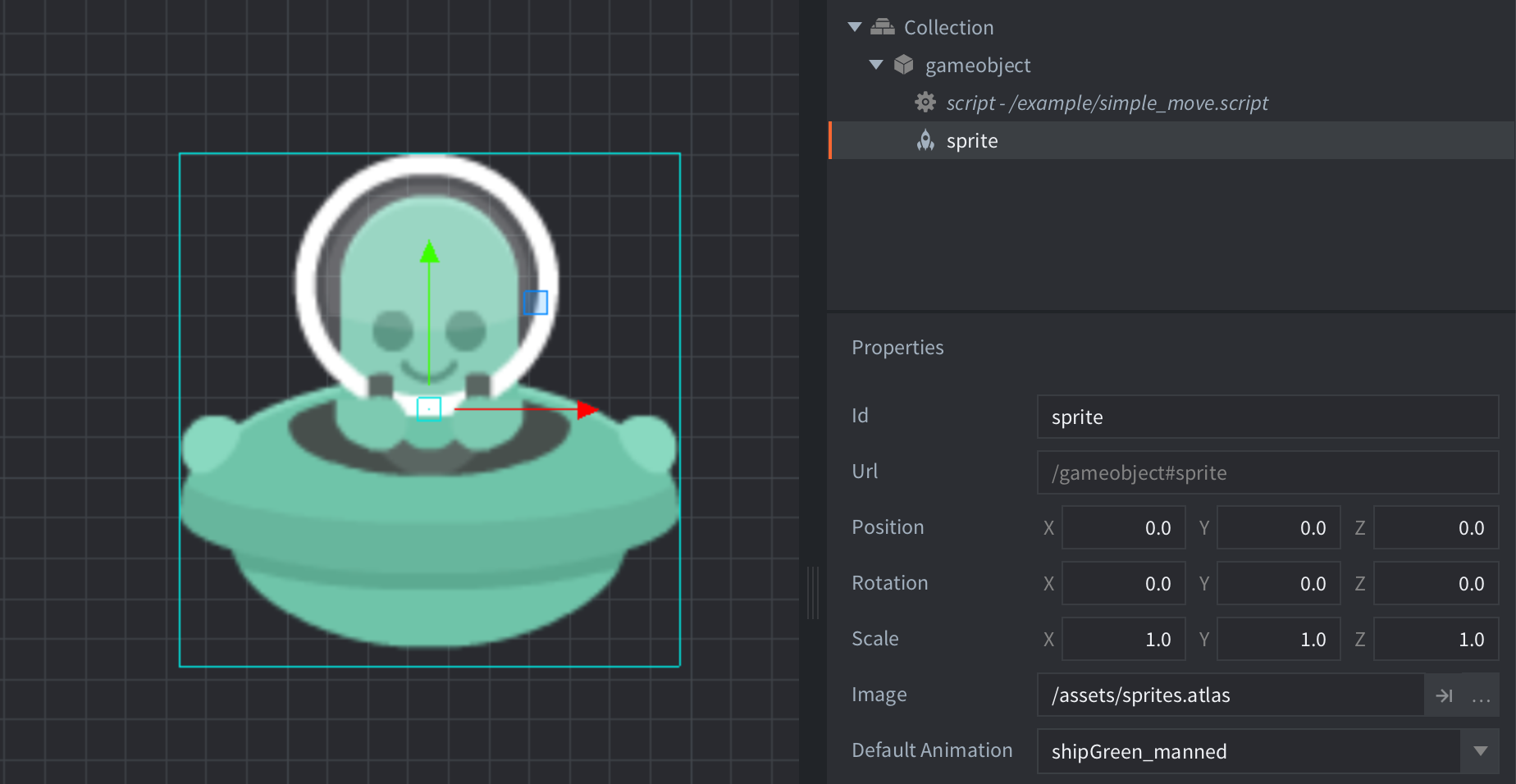#### Setup#### Scripts

simple_move.script

```function init(self)
self.center = vmath.vector3(360, 360, 0) -- <1>
self.speed = 2 -- <3>
self.t = 0 -- <4>
end

function update(self, dt)
self.t = self.t + dt -- <5>
local dx = math.sin(self.t * self.speed) * self.radius -- <6>
local dy = math.cos(self.t * self.speed) * self.radius
local pos = vmath.vector3() -- <7>
pos.x = self.center.x + dx -- <8>
pos.y = self.center.y + dy
go.set_position(pos) -- <9>
end

--[[
1. Store the center of rotation in the script instance (available through `self`).